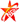您的位置：首页 >墨浪花>第十二期>教研阵地

# 求 同 存 异

—— 一道题引起的思考

(1)求f(x)的单调区间；

(2)令g(x)= -x2+2x+k，若对[0,2]，均[0,2]，使得f(x1)2)，求实数k的取值范围。

[解析](1)，有f(x)在x=2处的切线方程为y=9x-14，

∴则解得

∴f(x)= x3-3x+2，则=3x2-3=3(x+1)(x-1).

(2)对[0,2]，均[0,2]，使得f(x1)2)成立，即f(x)maxmax.

∴函数f(x)在[0,2]上的最大值f(x)max=f(2)=4.

∴函数g(x)在[0,2]上的最大值g(x)max =g(1)=k+1.

∵有f(x)maxmax，则43.

[点评]本题的难点在于第(2)小题中对“若对[0,2]，均[0,2]，使得f(x1)2)”这个已知条件的理解，很多同学是丈二和尚摸不着头脑，主要是理不清“任意”与“存在”之间的内在关系。我们不妨将这个已知条件从两个层面来分析，（1）先将g(x2)看做已知量，对[0,2]有f(x)2)等价于f(x)max2)，(2)再将f(x1)看做已知量，若[0,2]，使f(x1)1)max通过从这两个层面的分析不难理解出：若对[0,2]，均[0,2]，使得f(x1)2)f(x)maxmax.

在原题的基础上，现对第(2)小题进行以下三种变式，以帮助学生对此类题型更深刻的理解。

[解析] 对[0,2]和[0,2]，使得f(x1)2)f(x)maxmin

∴函数f(x)在[0,2]上的最大值f(x)max=f(2)=4

∴函数g(x)在[0,2]上的最小值g(x)min=g(0)=g(2)=k

∵有f(x)maxmin则4

[解析] 对[0,2]和[0,2]，使得f(x1)2)f(x)minmin

[解析]若[0,2]且[0,2]，使得f(x1)2)f(x)minmax

（祝志好老师  编审）【打印正文】

1、本网所有内容，凡注明"来源：六安新闻网"的所有文字、图片和音视频资料，版权均属六安新闻网所有。
2、本站版权所有内容纯属作者个人观点，仅供参考，欢迎转载，请务必注明出处：[六安新闻网]